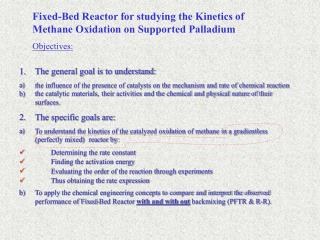DownloadDownload PresentationFixed-Bed Reactor for studying the Kinetics of Methane Oxidation on Supported Palladium

# Fixed-Bed Reactor for studying the Kinetics of Methane Oxidation on Supported Palladium

Download Presentation## Fixed-Bed Reactor for studying the Kinetics of Methane Oxidation on Supported Palladium

- - - - - - - - - - - - - - - - - - - - - - - - - - - E N D - - - - - - - - - - - - - - - - - - - - - - - - - - -
##### Presentation Transcript

1. Fixed-Bed Reactor for studying the Kinetics of Methane Oxidation on Supported Palladium Objectives: • The general goal is to understand: • the influence of the presence of catalysts on the mechanism and rate of chemical reaction • the catalytic materials, their activities and the chemical and physical nature of their surfaces. • The specific goals are: • To understand the kinetics of the catalyzed oxidation of methane in a gradientless (perfectly mixed) reactor by: • Determining the rate constant • Finding the activation energy • Evaluating the order of the reaction through experiments • Thus obtaining the rate expression b) To apply the chemical engineering concepts to compare and interpret the observed performance of Fixed-Bed Reactor with and without backmixing (PFTR & R-R).

2. (1) Theoretical backgrounds The oxidation of Methane is envisioned as a single step reaction The global rate of a solid catalyzed reaction is normally expressed as: Moles of reactant consumed per unit time per unit mass of catalyst (or per unit volume of the reactor containing the catalyst) Tr is the reference temperature, taken to be 250C

3. How to measure the global reaction rate [r(C,T)]? We need to start from species mass balance equation for particular reactor. accumulation = flow in - flow out +generation by reaction (2) PFR PFR-R

4. Equations For a Gradientless Reactor (CSTR or PFRR) Gradienless reactor(Perfectly mixed) in which all physical rate limitations are neglected, and uniform temperature and constant pressure (3) (4) CSTR PFRR PFR-R Where m is the weight of the catalyst (5) Where X, the fractional conversion of the reactant, is given by (6)

5. Substitution from eq (1) for r(C,T) and from eq (5) for Cr casts eq (4) in the following form, which relates the reactant (Methane) conversion to rate constant and operating conditions (7)

6. Co C x Co C An Ideal Plug Flow Reactor This model can be described by the following differential equation for the methane material balance x (8) where x is the axial position, made dimensionless by the reactor length. The initial condition for Eq 8. is the concentration at x = 0. (9) For the constant temperature case, Eq. (8) is readily integrated to give the concentration as a function of position. Application of Eq. (9) and substitution of the fractional conversion x (now the conversion at x = 1) from Eq. (6) leads to the following equation for the reactor conversion. (6) (10)

7. If the reaction order (n) is 1/2then the fractional conversion of Methane will be in the following form 11

8. The Experimental Setup

9. Experiment Procedure First session Run the reactor with zero recycle (PFR) Second session Run the reactor with recycle flow above 7 liters/min Third session Run the reactor as in the second session with different Methane concentration. P.S. Keep same feed composition in the first and second sessions

10. Feed gases are: Oxygen Flows at rate of(30ml/min), Mixture of 2% (by volume) Methane in Helium Flows at different rates (30,25, 20, or 16 ml/min) Helium for balance Total flow to the reactor is 100ml/min

11. Activation energy without catalyst Activation energy with catalyst Product Reactant Progress of the reaction

12. R-R PFTR x T R-R T1 T2 R-R x 1<n<1 r T5 n & k (T) Cor Cr Tr/T How to analyze our data Apply the data on Eq. (6) to calculate experimental conversion (x) for PFR and R-PFR. Plot x vs T (reaction temperatures) for both reactors for same initial concentration of CH4. 2. To evaluate the reaction order (use the experimental data of R-PFR): • Construct curves by plotting x versus Cor (initial concentration of CH4) for varying temperatures, see if the slopes of these lines show negative, zero or positive values. • Use Eq. (5) [r(C,T)=qr/mCorx] to calculate r(C,T) (reaction rate) as a function of reaction temperature for all initial concentrations of methane. • Plot rate data versus C (i.e., CrTr/T) for varying temperatures on logarithmic coordinates. According to Eq. (1) the straight lines should have slope equal to the reaction order n and the intercept equal to k(T).

13. R-R R-R Slope = -E/R Intercept=ko r T 1/T R-R If n=1/2 Ln k x(Cor/1-x)1/2 T 3. Use k(T) values and corresponding temperatures to create an Arrhenius plot. The ko and E/R (activation energy) values can be find from the intercept and the slope of the straight line. To test the kinetic model, Eq. (7) and, n=1/2, ko and E/R values can be used to calculate the conversion x as a function of the reactor temperature. Plot x(Cor/1-x)1/2 vs T of observed and predicted . See if all data points fall on same line, if so then the model is correct. 5. To test the consequences of varying the initial concentrations of methane on the reaction rate, plot r versus T for various Cor.

14. 6. Use Eq. (11) to calculate conversion x for PFTR. R-R PFTR x T 7. Plot experimental and calculated conversion values versus T for both reactors (P.S. for same initial feed concentration).

15. R-R PFTR x T T1 R-R T2 R-R r x 1<n<1 T5 n & k (T) Cor CrTr/T

16. R-R Slope = -E/R Intercept=ko Ln k 1/T R-R If n=1/2 x(Cor/1-x)1/2 T

17. R-R r T R-R PFTR x T